## Example Questions

2 Next →

### Example Question #7 : How To Find The Equation Of A Parallel Line

Which of these formulas could be a formula for a line perpendicular to the line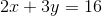?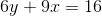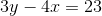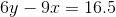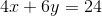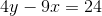Explanation:

This is a two-step problem. First, the slope of the original line needs to be found. The slope will be represented by "" when the line is in-intercept form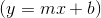.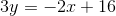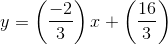So the slope of the original line is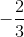. A line with perpendicular slope will have a slope that is the inverse reciprocal of the original. So in this case, the slope would be. The second step is finding which line will give you that slope. For the correct answer, we find the following: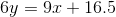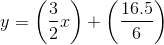So, the slope is, and this line is perpendicular to the original.

### Example Question #11 : Lines

Which of the following equations is parallel to: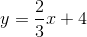and goes through the point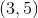?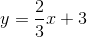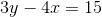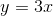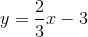Explanation:

Step 1: We need to define what a parallel line is. A parallel line has the same slope as the line given in the problem. Parallel lines never intersect, which tells us that the y-intercepts of the two equations are different.

Step 2: We need to identify the slope of the line given to us. The slope is always located in front of the.

The slope in the equation is.

Step 3: If we said that a parallel line has the same slope as the given line in the equation, the slope of the parallel equation is also.

Step 4. We need to write the equation of the parallel line in slope-intercept form:. We need to write b for the intercept because it has changed.

The equation is: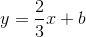Step 5: We will use the pointwhereand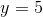. We need to substitute these values of x and y into the equation in step 4 and find the value of b.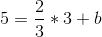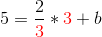The numbers in red will cancel out when I multiply.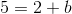To find b, subtract 2 to the other side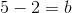Step 6: We put all of the parts together and make the final equation of the parallel line:

The final equation is:2 Next →